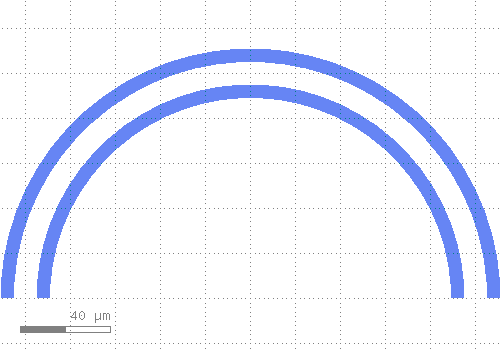# kqcircuits.elements.waveguide_coplanar_curved¶

kqcircuits.elements.waveguide_coplanar_curved.arc(r, start, stop, n)[source]

Returns list of points of an arc

Parameters

• start – begin angle in radians

• stop – end angle in radians

• n – number of corners in full circle

class kqcircuits.elements.waveguide_coplanar_curved.WaveguideCoplanarCurved[source]

The PCell declaration of a curved segment of a coplanar waveguide.

Coordinate origin is left at the center of the arc.

coerce_parameters_impl()[source]

default implementation

build()[source]

Child classes re-define this method to build the PCell.

static create_curve_arcs(elem, angle)[source]

Creates arcs of points for a curved waveguide.

Parameters
• elem – Element from which the waveguide parameters for the arc are taken

• angle (double) – angle of the curved waveguide

Returns

A tuple consisting of lists of points, each list representing one of the arcs. (left_gap_inner, left_gap_outer, right_gap_inner, right_gap_outer, left_protection, right_protection, annotation)

static produce_curve_termination(elem, angle, term_len, trans, face_index=0, opp_face_index=1)[source]

Produces termination for a curved waveguide.

The termination consists of a rectangular polygon in the metal gap layer, and grid avoidance around it. The termination is placed at the position where a curved waveguide with alpha=angle and trans=trans would end.

Parameters
• elem – Element from which the waveguide parameters for the termination are taken

• angle (double) – angle of the curved waveguide

• term_len (double) – termination length, assumed positive

• trans (DTrans) – transformation applied to the termination

• face_index (int) – face index of the face in elem where the termination is created

• opp_face_index (int) – face index of the opposite face

PCell parameters:

• alpha (Double) - Curve angle (rad), default=`3.141592653589793`

• length (Double) - Actual length, default=`0`, unit=`μm`

• add_metal (Boolean) - Add trace in base metal addition too, default=`False`

• ground_grid_in_trace (Boolean) - Add ground grid also to the waveguide, default=`False`